Ministério da Ciência, Tecnologia, Inovações e Comunicações CBPFIndex CENTRO BRASILEIRO DE PESQUISAS FÍSICAS11/12/2023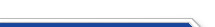Usuário: Senha: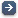Crie sua conta aqui · Busca rápida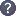Procurar por: Publicações Projetos EventosIr para a busca avançada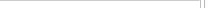Notas de Física
 First quantization of braided Majorana fermions Data do cadastro: 15/03/2022 Publicação/Divulgação: 15/03/2022 Resp. pelo cadastro: Status atual: Publicado Ano da publicação: 2022 Descrição: A ${\mathbb Z}_2$-graded qubit represents an even (bosonic) vacuum state" and an odd, excited, Majorana fermion state. The multiparticle sectors of $N$, braided, indistinguishable Majorana fermions are constructed via first quantization. The framework is that of a graded Hopf algebra endowed with a braided tensor product. The Hopf algebra is ${\cal U}({\mathfrak {gl}}(1|1))$, the Universal Enveloping Algebra of the ${\mathfrak{gl}}(1|1)$ superalgebra. A $4\times 4$ braiding matrix $B_t$ defines the braided tensor product. $B_t$, which is related to the $R$-matrix of the Alexander-Conway polynomial, depends on the braiding parameter $t$ belonging to the punctured plane ($t\in {\mathbb C}^\ast$); the ordinary antisymmetry property of fermions is recovered for $t=1$. \\ For each $N$, the graded dimension $m|n$ of the graded multiparticle Hilbert space is computed. Besides the generic case, truncations occur when $t$ coincides with certain roots of unity which appear as solutions of an ordered set of polynomial equations. The roots of unity are organized into levels which specify the maximal number of allowed braided Majorana fermions in a multiparticle sector. \\ By taking into account that the even/odd sectors in a ${\mathbb Z}_2$-graded Hilbert space are superselected, a nontrivial braiding with $t\neq 1$ is essential to produce a nontrivial Hilbert space described by qubits, qutrits, etc., since at $t=1$ the $N$-particle vacuum and the antisymmetrized excited state encode the same information carried by a classical $1$-bit. Número: CBPF-NF-002/22 Autores: F. Toppan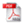Download do PDF (1.433 KB)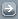Todos EventosTodos PublicaçõesTodasArtigos de Div. CientíficaArtigos em Revistas Nac.Artigos em Revistas Int.Artigos em Eventos Nac.Artigos em Eventos Int.Livros InteirosCapítulos de LivroPropriedades IntelectuaisÁudioPatentesProgramas de computadorModelos de utilidadeMarcasOutras prop. intel.TecnologiasKnow-howServiços tecnológicosTecnologias inovadorasTeses de DoutoradoDissertações de MestradoProjetos de GraduaçãoCiência e SociedadeDocumentos HistóricosMonografiasNotas de FísicaNotas TécnicasOutras Produções
 · Principal    |    CBPF    |    · Copyright 2004 - CBPF. Rio de Janeiro/BrasilCentro Brasileiro de Pesquisas Físicasv 1.1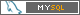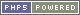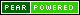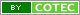Pï¿½g. gerada em 0.01 s Total de consultas SQL: 16